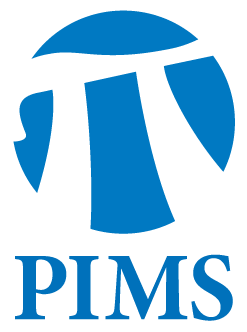Probability Seminar: Juan Souto

• Date: 03/21/2012
• Time: 15:00
Lecturer(s):
Juan Souto
Location:

University of British Columbia

Topic:

Distributional limits of Riemannian manifolds and graphs with sublinear genus growth

Description:

Abstract

Studying Gromov-Hausdorff limits of sequences of Riemannian manifolds
$(M_i)$ satisfying suitable conditions on their local geometry is an
extremely fruitful idea. However, in the most interesting case that the
diameter of $M_i$ grows without bounds, one is forced to choose base
points $p_i\in M_i$ and consider limits of the pointed spaces
$(M_i,p_i)$ in the pointed Gromov-Hausdorff topology. The choice of the
base points $p_i$ influences enormously the obtained limits. Benjamini
and Schramm introduced the notion of distributional limit of a sequence
of graphs; this basically amounts to "choosing the base point by
random". In this talk I will describe the distributional limits of
sequences $(M_i)$ of manifolds with uniformly pinched curvature and
satisfying a certain condition of quasi-conformal nature. I will also
explain how these results yield a modest extension of Benjamini's and
Schramm's original result. This is joint work with Hossein Namazi and
Pekka Pankka.

Other Information:

Location: WMAX 110